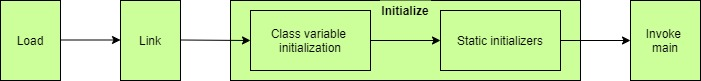## 1. Introduction

In this tutorial, we'll explore the static variable initialization process. The Java Virtual Machine (JVM) follows this process during class loading.

## 2. Initialization Process

At a high level, the JVM performs the following steps:First, the class is loaded and linked. Then, the “initialize” phase of this process processes the static variable initialization. Finally, the main method associated with the class is called.

In the next section, we'll look at class variable initialization.

## 3. Class Variable

In Java, static variables are also called class variables. That is, they belong to a class and not a particular instance. As a result, class initialization will initialize static variables.

In contrast, a class's instance will initialize the instance variables (non-static variables). All the instances of a class share the class's static variables.

Let's take an example of class StaticVariableDemo:

``````public class StaticVariableDemo {
public static int i;
public static int j = 20;

public StaticVariableDemo() {}
}``````

First, the JVM creates a Class object for the class StaticVariableDemo. Next, the static field initializers assign a meaningful default value to the static fields. In our example above, the class variable i is first initialized with an int default value of zero.

The textual order applies to static fields. First, i will initialize and then j will be initialized. After that, the class and its static members will be visible to other classes.

## 4. Variable in a Static Block

Let's take another example:

``````public class StaticVariableDemo {
public static int z;

static {
z = 30;
}
public StaticVariableDemo() {}
}``````

In this case, the variable initialization will be in sequence. For instance, the JVM initially assigns variable z to a default int value of 0. Then, in the static block, it is changed to 30.

## 5. Variable in a Static Nested Class

Finally, let's take an example of the nested class inside the outer StaticVariableDemo class:

``````public class StaticVariableDemo {
public StaticVariableDemo() {}

static class Nested {
public static String nestedClassStaticVariable = "test";
}
}``````

In this case, the class StaticVariableDemo loads the Nested class. It'll initialize the static variable nestedClassStaticVariable.

## 6. Conclusion

In this short article, we have briefly explained the static variable initialization. For further details, check the Java Language Specification.

As always, the code snippets are available over on GitHub.

### Get started with Spring 5 and Spring Boot 2, through the Learn Spring course:

>> CHECK OUT THE COURSE